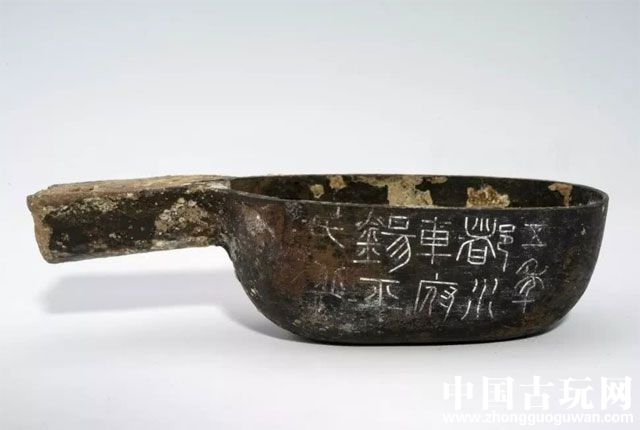​一、汉代

1 石 = 四钧 = 29760 克

1 钧 = 三十斤 = 7440 克

1 斤 = 16 两 = 248 克= 液体250 毫升

1 两 = 24 铢 = 15.625 克

1 圭= 0.5 克

1 撮 = 2 克

1 方寸匙 = 金石类2.74 克= 药末约2 克= 草木类药末约1 克

1 斛 = 10 斗 = 20000 毫升

1 斗 = 10 升 = 2000 毫升

1 升 = 10 合 = 200 毫升

1 合 = 2 龠 = 20 毫升

1 龠 = 5 撮 = 10 毫升

1 撮 = 4 圭 = 2 毫升

1 圭 = 0.5 毫升

“石”：读shí，后来读dàn

“龠”：读yuè

1 引 = 10 丈 = 2310 厘米

1 丈 = 10 尺 = 231 厘米

1 尺 = 10 寸 = 23.1 厘米

1 寸 = 10 分 = 2.31 厘米

1 分 = 0.231 厘米

------------

1 石 = 120斤 = 70800克

1 斤 = 16 两 = 590 克

1 两 = 36.9克

1 钱=10分=3.69 克

1 分=0.369克

1石=2斛=100000毫升

1 斛 =5斗 = 50000 毫升

1 斗 = 10 升 = 1000 毫升

1 升 = 10 合 = 1000 毫升

1 合 = 10 毫升

1 引 = 10 丈 =355厘米

1 丈 = 10 尺 = 35.5 厘米

1 尺 = 10 寸 = 3.55厘米

1 寸 = 10 分 = 2.31 厘米

1928年，中华民国政府公布度量衡法，规定采用“万国公制”为标准制，并暂设辅制“市用制”作为过渡，即1公尺为3市尺，1公升为1市升，1公斤为2市斤。改革后的市制适应民众习惯，又与公制换算简便，逐渐为民众接受，1949年后，市用制通行全国。1984年，国务院发布命令，采用以国际单位制为基础，同时选用一些非国际单位制单位的中华人民共和国法定计量单位(简称法定单位)。自1991年1月1日起，法定单位成为中国唯一合法的计量单位。

1吨t=1000kg公斤

1公斤kg=1000克g

1公两=100克g